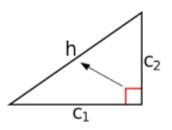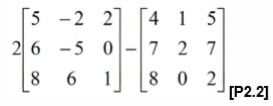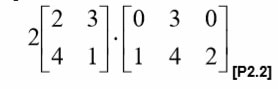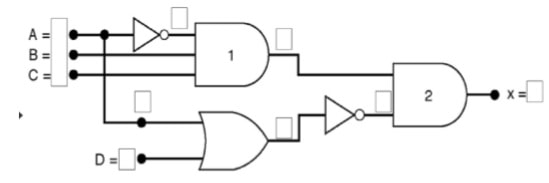# BTEC HND in Computing and Systems Development Unit 23

```{`
Programme title BTEC HND in Computing Systems Development
Unit number and title 23 Mathematics for Software Development (L4)
Assignment number & title 1 of 1 Mathematics for Software Development
`}```

## Assignment:

### Case Assignment/ Scenario

Scenario (all names and details appearing in the scenario below are entirely fictional)

You are employed in a Games development company as a junior software engineer. As part of your role you will need to produce Pseudo-code/Flowcharts for a number of mathematical operations and functions. You are also required to write code and design programming solutions for such operations and functions.

### LO1: Understand core mathematical skills for software engineers

```{`
1.1  design a programming solution to a given algebraic problem
1.2  design a programming solution to a given geometric problem
1.3 implement code that presents a range of vectors
`}```

1. Answer the following algebraic questions:

1. Determine the solution for the linear equation 6x-b=c. when b = 15 and c = 45. Draw the above linear equation in a graph. Design a program using c# programming language that will find the value of x for any value of b and c, your program must allow the user to input the values of b and c. [P1.1]
2. Given a quadratic equation ax2 + bx + c = 0, find the values of x in the above equation when a is equal to 1, b is equal to -9 and c is equal to 20. Draw the graph of the equation and find the minimum/maximum points [P1.1]
3. Design a program to read in the coefficients a, b and c for any value of a, b, and c. The program should compute and display the roots if the roots are noncomplex. The program should display a message if the roots are complex. [M1]

2. Pythagoras' theorem, is a fundamental relation in Euclidean geometry among the three sides of a right triangle. Design and implement a programming solution for the following geometric and trigonometric questions:

1. A flagpole broke in a storm. 7 meters are still sticking straight out of the ground, where it snapped, but the remaining piece has hinged over and touches the ground at a point 24 meters away horizontally. How tall was the flagpole before it broke?[P1.2]
2. Find the Hypotenuse (h) when C1 is 40 cm and C2 is 20 cm in the diagram below and find the area of the shape. [P1.2]3. You are required to design a program (using pseudocode or flowchart diagram) that will calculate the volume of a cuboid, rectangle and a square considering user inputs.[ M1]

3. Implement the programming code (as specified) to solve the following vectors questions:

1. Two forces with magnitudes of 15 Newton and 35 Newton are applied to an object. The magnitude of the resultant is 28 Newton. Find the measurement of the angle between the resultant vector and the vector of the 15 Newton force to the nearest whole degree.[P1.3]
2. You are required to design a c# program that will add and subtract two generic vectors: a = (x,y,z) and b = (x, y, z). The values of x, y and z should be input by the user and the outcome of the result displayed to the user. [D1]
3. Design a c# program were the user will input the coordinates of a 2 dimensional (2D) vector and rotate it at an angle of 50. The program must output the new coordinates of the rotated vector. [D1]
• To achieve M1 the student should show that the validity of result has been evaluated using defined criteria when finding the areas and volumes in 1(a). The student should also demonstrate an effective approach when calculating the area of the shape in 2(c) and will have met the deadline to submit the tasks and achieve the unit assessment criteria.
• To achieve D1 the student needs to demonstrate how you have validated the output of the program (e.g. new position of the vector after rotation) in 3(c).

### LO2: Understand the application of algebraic concepts

```{`
2.1 explain how relations link to technologies used in programming
2.2 design a programming solution to solve a given matrix manipulation
`}```

1.

1. Explain in your own words what a universal relation is. In your explanation you should include all types of universal relations (Reflexive, Symmetric, Transitive and Equivalence). ? [P2.1]
2. Explain what a function is? Show the pictorial representation of a function.  [P2.1]
3. Consider R= &lbrace(x,y)∈R2|x2+y2=1 &rbrace , is R
1. Reflexive
2. Symmetric?
3. Antisymmetric?
4. Transitive? [P2.1, M2]
4. Let: A = &lbrace a1,a2,a3,a4,a5 &rbrace ,B = &lbrace b1,b2,b3,b4,b5 &rbrace, f= &lbrace (a1,b2), (a2,b3), (a3,b3), (a4,b1), (a5,b4) &rbrace, S= &lbrace a1,a3 &rbrace
1. Draw a diagram for f
2. What is the: Domain, co-domain, range of f?
3. What is Image of S, f(S)? [P2.1][M2]

2.

1. Solve the following matrix subtraction:2. Write a program to find the solution of the problem in (a). [D2]
3. Multiply the following two matrices and show the resultant matrix:4. Design a pseudo code or flowchart diagram that demonstrates how to perform product or multiplication of two matrices. [P2.2, D2]
• To achieve M2 the student should apply a range of methods and techniques when producing the pseudocode for finding the domain and range.
• To achieve D2 the student should demonstrate that autonomy/independence has been demonstrated when solving the matrices problem and that activities have been managed. The student should submit assignment in time.

### LO3: Be able to apply the fundamentals of formal methods

```{`
3.1 discuss the application of set theory in computing
3.2 design a programming solution to a given propositional calculus problem
`}```

1.

1. Answer the following set related questions :
1. I. A= &lbrace -3, -2, -1, 0, 1, 2, 3 &rbrace write A in set form.
2. II. B = &lbrace 3,6,9,12 &rbrace write B is set form
3. III. If A = &lbrace 1, 2, 3, 4 &rbrace , B = &lbrace 2, 3, 4, 5 &rbrace. is A ⊆ B or B ⊆ A?
4. IV. Write the power set of: B = &lbrace y : y∈N and1≤ y ≤ 3 &rbrace [P 3.1]
2. Given that:
A = &lbracex : x is a even natural number less than or equal to 10 &rbrace B = &lbrace x : x is an odd natural number less than or equal to 10 &rbrace Find (i) A-B (ii) B-C (iii) is A-B=B-A? [P3.1]
3. In a className of 100 students, 35 like science and 45 like math. 10 like both. How many like either of them and how many like neither? [P3.1]
4. Design a C# program to find first n number of Fibonacci series. [M3]

2. Solve the following propositional calculus and logic problems:

1. Solve the following propositional problem S = (P ∨ Q) ∧ ¬(P ∧ Q ) and discuss the outcome. (hint: produce the truth table of the above problem)  [P3.2]
2. Determine the logical expression for the logic circuit below and then design and implement programming solution that will help you to find the truth table for the circuit. [P3.2, M3]3. Draw the circuit diagram of the following expression:[ ¬ symbol means a bar] X = ¬(A)BC ¬(A + D) [P3.2, M3]

To achieve M3 a range of methods of presentation must be used and technical language is accurately used when designing and providing the truth table for the circuit.

### LO4: Be able to apply statistical techniques to analyse data

```{`
4.1 design a programming solution to solve a given statistical analysis technique
`}```

1. Find the following two data sets of salary:

```{`
Set 1 £300, 300, 300, 940, 300, 300, 400, 300, 400, 450, 800, 450, 1050
Set 2 £300, 300, 940, 450, 400, 400, 300, 300, 1050, 300
`}```
1. Find mean, median, mode, range, standard deviation, first quartile and variance of the two data sets.[P4.1]
2. Design and implement a programming solution using C# for the question in a). [P4.1]
3. According to a study there is roughly 80% chance that a person aged 20 will be alive at age 65. Suppose that three people aged 20 are selected at random. Find the probability that  the number of people alive at age 65 will be:
1. Exactly two.
2. At most one.
3. At least one.

[Hint: Use binomial probability distribution formula]

2. Design a C# program to simulate 100 trials of a coin toss and draw the histogram. [D3]

To achieve D3 the student should demonstrate that ideas have been generated and decisions taken when designing the program to find the statistical values in Q1(c) and Q2.